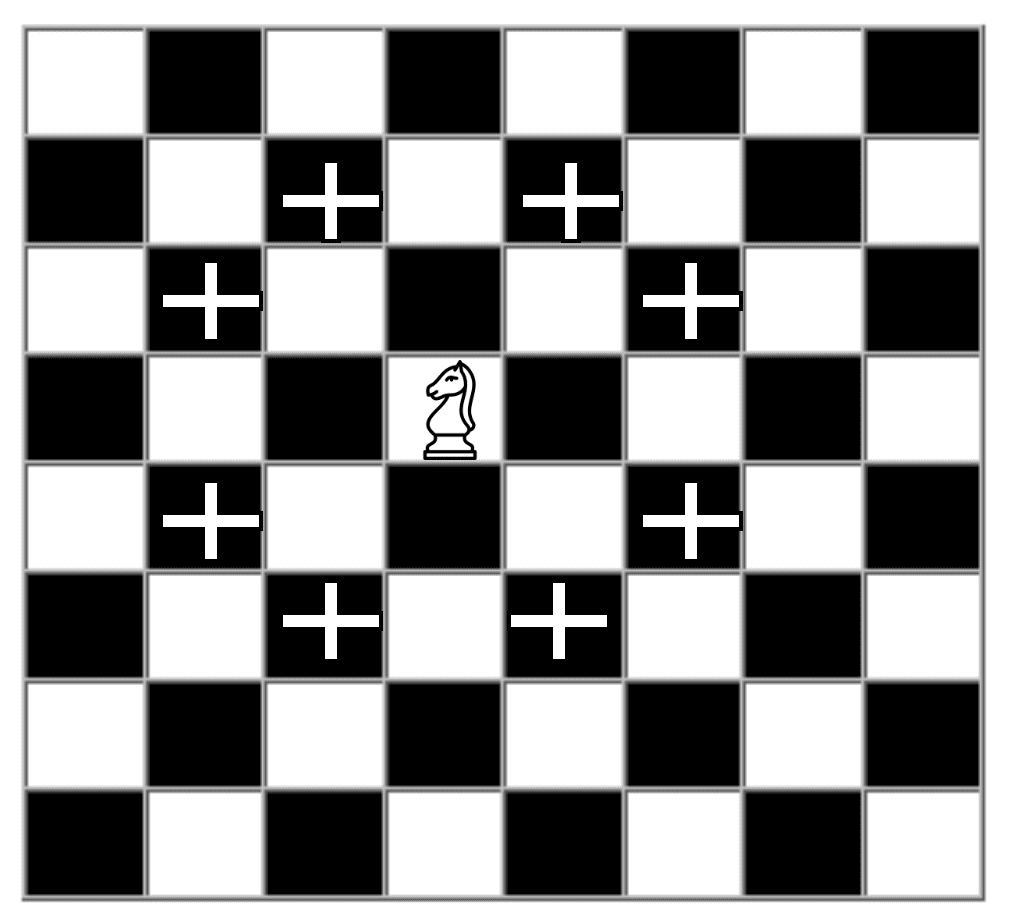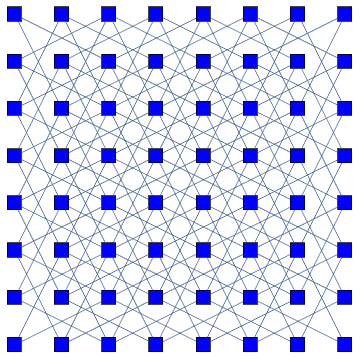## 1. Overview

In this tutorial, we’ll discuss the knight’s shortest path problem on a chessboard. Furthermore, we’ll explain the problem statement and provide an efficient solution.

## 2. Problem Statement

Given a standardchessboard, we want to find the minimum number of steps for a knight to reach a destination positionfrom a source position. Here, the source and destination position of the knight on a chessboard is known.

Now let’s take a look at the possible movements of a knight from a random position on a chessboard:On a chessboard, a knight can move two ways. It can either move one square horizontally and two squares vertically, or one square vertically and two squares horizontally. Hence, from a given position (X, Y), the possible moves are:Here, we’ve shown thepossible positions on a chessboard a knight can move from a given initial position. At any point in time, a knight can’t go out of the chessboard. Finally, if there is a situation that the knight can’t reach the destination from the given initial position, we’ll return.

## 3. Approach

In order to solve this problem, first, we’ll convert the chessboard to a knight’s graph:In a knight’s graph, each box on a chessboard denotes a vertex. Each vertex corresponds to the position of a knight on a chessboard. Each edge represents a legal move of a knight. Hence, we can apply any searching algorithm to find the shortest path from one point to another in order to solve the knight’s shortest path problem. We’re using Breadth-First Search (BFS) algorithm here.

Initially, we’ll position a knight randomly on a chessboard. From the initial point, we’ll aim to explore all possiblepositions. If all the possible positions which can be reached from the initial position are not visited, we enqueue this state into a queue. Moreover, we increase the number of moves byfrom the last state in the queue.

At each new position, we’ll check if the current position of the knight is the destination position or not. If the current position is not the destination, we’ll pop the current position from the queue and enqueue the possible positions a knight can move from the current position.

We’ll continue until we reach the destination position or explore all the possible positions on a chessboard.

## 4. Pseudocode

Let’s look at the pseudocode now:Here,andare two direction arrays that denote the change in the X-direction and the Y-direction. Furthermore, we created a queueand pushed the knight’s initial position.

Initially, we created an arrayto decide whether a given position on a chessboard is already explored or not.  In the beginning, we initialized it toin order to depict all the positions are unexplored. When we start the iteration, we make the knight’s initial position in thearray as.

Moreover, we used an arrayin order to store the number of steps required for a knight to reach the destination position. We run the algorithm until the queue is empty or the destination is reached.

To check whether a given position is inside the chessboard or not,function is defined. Here is the expansion of:Finally, let’s talk about the time complexity of the pseudocode. So the main complexity of this pseudocode is visiting the chessboard cells to reach the destination. If the dimension of the given chessboard is, the time complexity of this algorithm will be.

## 5. Conclusion

In this tutorial, we discussed the knight’s shortest path problem on a chessboard. We presented a BFS based approach for solving this problem with pseudocode.